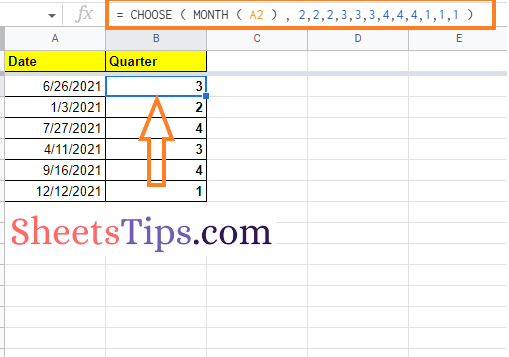# How To Extract Quarter in Google Sheets? – Quarter Function in Google Sheets

Getting a quarter value from the given date can be quite tricky in Google Sheets since it does not have any inbuilt functions. However, with the help of a few in-built functions and formulas, we can easily extract the quarter value in Google Sheets. If you are looking to extract the month from the given dates, then this page is for you.

On this page, let us discuss various methods through which we can extract the month using Google Sheets tips and tricks. Read further to find out more.

## How To Extract Quarters Using The MONTH Function in Google Sheets?

The MONTH function is one of the inbuilt functions in Google Sheets. The steps to extract a quarter using the MONTH function in the spreadsheet are explained below.

• 2nd Step: Move to the cells where you want to extract quarters using the MONTH function.
• 3rd Step: Now enter the formula “= CHOOSE (MONTH (A2)), 1,1,1,2,2,3,3,4,4,4)“.
• 4th Step: Press the Enter key and you will find the results as shown in the image below.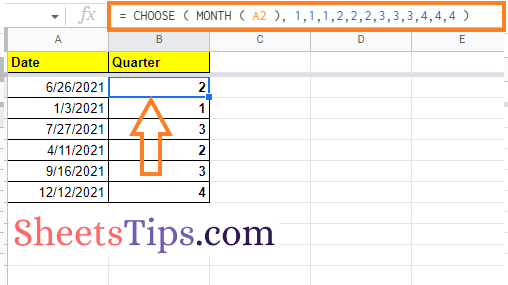Now drag the formula applied cell to other parts of the cell to apply the same formula and get the same results.

### How to Get a Quarter Using the Roundup Function?

Google Sheets allows its users to extract the quarter-month from the given dataset using the ROUNDUP function. The steps to get this done in Google Sheets are explained below.

• 2nd Step: Move to the cells where you want to extract the quarter from the given dates.
• 3rd Step: Enter the formula “= ROUNDUP (MONTH (A2)/3, 0)“.
• 4th Step: Press the “Return” key and you will find the results as shown in the image given below.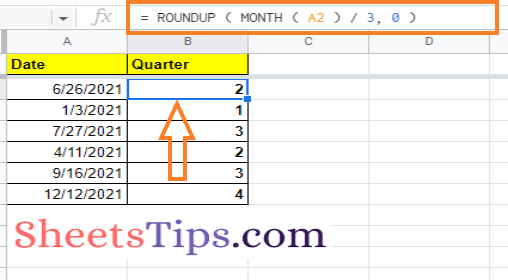To get the same results across other cells, just drag the formula-applied cell to other parts of the cell in Google Sheets.

### How to Extract Quarter in Google Sheets Using the Query Function?

Another easy method to extract the quarter function in Google Sheets is by using the QUERY function. The steps to extract quarter using the QUERY function are given below.

• 2nd Step: Now on the homepage, click on the cell where you want to get the quarter from the dates.
• 3rd Step: Here enter the formula “=QUERY (A2:A, “SELECT QUARTER (A) LABEL QUARTER (A) ‘Quarter'”)“.
• 4th Step: Press the “Enter” key and you will find the results as shown in the image below.
• 5th Step: Drag the formula applied cell to other parts of the cell to display the same results.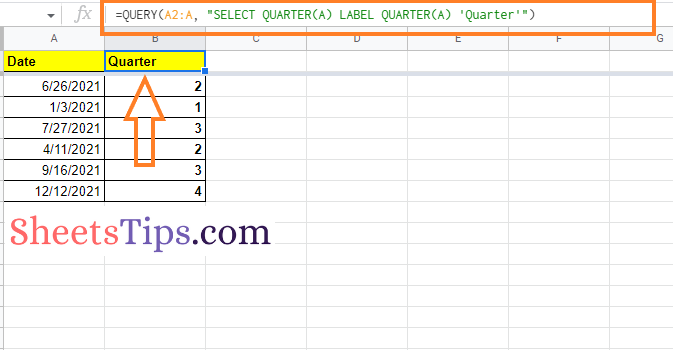### How to Display Quarters in Google Sheets as Q1, Q2, Q3, and Q4?

Google Sheets also lets its users display the quarter as of Q1, Q2, Q3, or Q4 using the CHOOSE function. The detailed steps of how to do this in Google Sheets are explained below.

• 1st Step: The first step is to launch Google Sheets on your device.
• 2nd Step: Go back to the homepage and click on the cell where you want the quarter to be calculated from the dates.
• 3rd Step: Type in “= “Q” & CHOOSE ( MONTH ( A2 ), 1,1,1,2,2,2,3,3,3,4,4,4 )” in the third step.
• 4th Step: Press the “Enter” key, and the results will appear as in the image below.
• 5th Step: To apply the same formula to other cells, drag the formula-applied cell to other areas of the cell.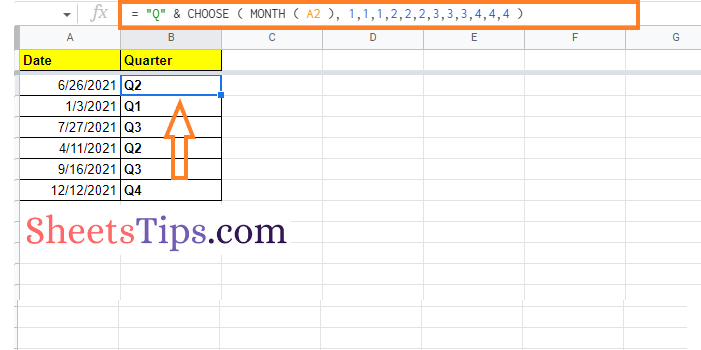### How to Get a Quarter for a Non-Standard Calendar Year?

Many countries follow different quarters. For example, some countries prefer to start their fiscal year in July, while others prefer to start their fiscal year in January. And to help users in those situations, Google Sheets allows spreadsheet users to tweak the non-standard calendar years. Let us understand how to do this in a spreadsheet.

For example, let us consider a country that is starting its fiscal year in July, and the detailed steps for tweaking the formula for the same are outlined below: